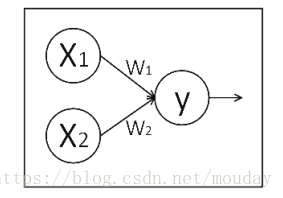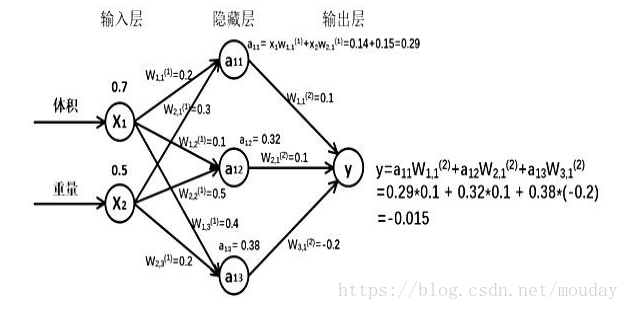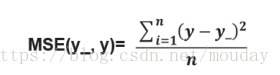# 人工智能实践Tensorflow笔记：Tensorflow框架-3

0 阶张量称作 标量scalar

1 阶张量称作 向量vector []

2 阶张量称作 矩阵matrix [[]]

Tensorflow 的数据类型有 tf.float32、tf.int32 等。函数 说明 tf.random_normal() 生成正态分布随机数 tf.truncated_normal() 生成去掉过大偏离点的正态分布随机数 tf.random_uniform() 生成均匀分布随机数 tf.zeros 表示生成全 0 数组 tf.ones 表示生成全 1 数组 tf.fill 表示生成全定值数组 tf.constant 表示生成直接给定值的数组

1、准备数据集，提取特征，作为输入喂给神经网络（Neural Network，NN）

2、搭建 NN 结构，从输入到输出（先搭建计算图，再用会话执行）

（ NN 前向传播算法 计算输出）

3、大量特征数据喂给 NN，迭代优化 NN 参数

（ NN 反向传播算法 优化参数训练模型）

4、使用训练好的模型预测和分类W 前节点编号，后节点编号(层数)

loss_mse = tf.reduce_mean(tf.square(y_ - y))train_step=tf.train.MomentumOptimizer(learning_rate, momentum).minimize(loss)

linux:

vim ~/.vimrc 写入：

set ts=4 表示使 Tab 键等效为 4 个空格

set nu 表示使 vim 显示行号 nu 是 number 缩写

“提示 warning”，是因为有的电脑可以支持加速指令，

“提示 warning”暂时屏蔽掉。主目录下的 bashrc 文件，

“0”（显示所有信息）

“1”（不显示 info），

“2”代表不显示 warning，

“3”代表不显示 error。一般不建议设置成 3

source 命令用于重新执行修改的初始化文件

`# -*- coding: utf-8 -*-  # @File    : 搭建神经网络八股.py # @Date    : 2018-06-02  # 搭建神经网络的八股 # 准备工作 -> 前向传播 -> 反向传播 -> 循环迭代  # 1、导入模块，生成模拟数据集； import tensorflow as tf import numpy as np  BACH_SIZE = 8 SEED = 23455  # 基于随机数产生 32行2列的随机数 rng = np.random.RandomState(SEED) X = rng.rand(32, 2)  #  随机数组, 浮点数，[0, 1)均匀分布  # 输入数据集的标签（正确答案）x0+x11   x0+x1>=1 -> 0 Y = [[int(x0 + x1 < 1)] for (x0, x1) in X] print("X: \n%s"%X) print("Y: \n%s"%Y)  # 2、定义神经网络的输入、参数和输出，定义前向传播过程； x = tf.placeholder(tf.float32, shape=(None, 2)) y_ = tf.placeholder(tf.float32, shape=(None, 1))  # 生成正态分布随机数，形状两行三列，标准差是 1，随机种子是 1 w1 = tf.Variable(tf.random_normal(shape=[2, 3], stddev=1, seed=1)) w2 = tf.Variable(tf.random_normal(shape=[3, 1], stddev=1, seed=1))  a = tf.matmul(x, w1) y = tf.matmul(a, w2)  # 3、定义损失函数及反向传播方法 # 均方误差 loss = tf.reduce_mean(tf.square(y - y_)) # 随机梯度下降算法，使参数沿着梯度的反方向，即总损失减小的方向移动，实现更新参数。 train_step = tf.train.GradientDescentOptimizer(0.001).minimize(loss)  # 4、生成会话，训练 STEPS 轮 with tf.Session() as session:  init_option = tf.global_variables_initializer()  session.run(init_option)   # 输出未经训练的参数值  print("w1: \n", session.run(w1))  print("w2 \n", session.run(w2))  print("\n")   # 训练模型  STEPS = 3000  for i in range(STEPS):  start = (i*BACH_SIZE)%32  end = start + BACH_SIZE  session.run(train_step, feed_dict={x: X[start: end], y_: Y[start: end]})  if i %500 == 0:  total_loss = session.run(loss, feed_dict={x: X, y_:Y})  print("ssetp %d, loss %s"% (i, total_loss))   # 训练后的取值  print("w1\n", session.run(w1))  print("w2\n", session.run(w2)) """  由神经网络的实现结果，我们可以看出，总共训练 3000 轮，每轮从 X 的数据集 和 Y 的标签中抽取相对应的从 start 开始到 end 结束个特征值和标签，喂入神经 网络，用 sess.run 求出 loss，每 500 轮打印一次 loss 值。经过 3000 轮后，我 们打印出最终训练好的参数 w1、w2。 """`